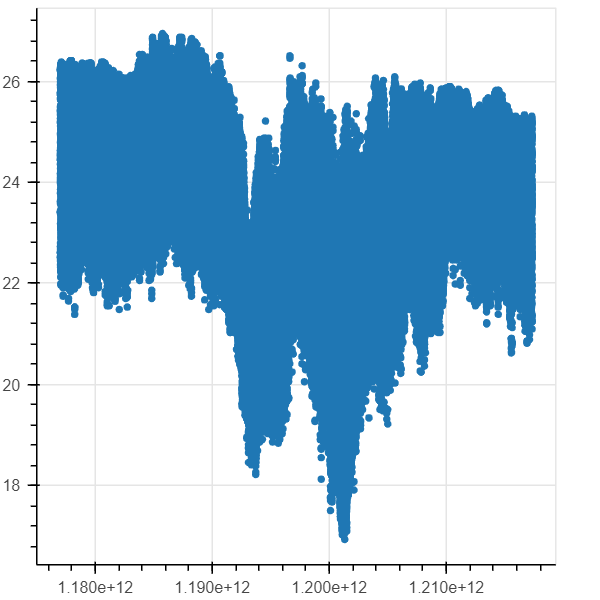# Multiindex, netCDF DataSource

Hey,

i tried to visualize a netCDF Datasource but i cant get the transformation from xarray via pandad to bokeh datasource to work. The different dimensions are turned into a MultiIndex Column and i cant figure out how to use parts of this column with bokeh.

Here are parts of my code. Do you have any examples for me?

from bokeh.plotting import figure, output_file, show
from bokeh.models import ColumnDataSource

import pandas
import xarray as xr

df =first.to_dataframe()

df.drop(columns=[“INST_MFGR”,“INST_MODEL”,“INST_SN”], inplace=True)

# Dropping those columns because they contain Strings: b’INST_123’

src = ColumnDataSource(df)

print(src.column_names)
`# OUT: ['INST', 'TEMP', 'DEPTH_LATITUDE_LONGITUDE_TIME'] `

p = figure(plot_width=400, plot_height=400)

p.circle(x=‘TIME’, y=‘TEMP’, source=src)

# show the results

show§

``

You can do this:
df =first.to_dataframe().reset_index()

and it will produce:···

Le dimanche 25 novembre 2018 04:37:50 UTC+1, [email protected] a écrit :

Hey,

i tried to visualize a netCDF Datasource but i cant get the transformation from xarray via pandad to bokeh datasource to work. The different dimensions are turned into a MultiIndex Column and i cant figure out how to use parts of this column with bokeh.

Here are parts of my code. Do you have any examples for me?

from bokeh.plotting import figure, output_file, show
from bokeh.models import ColumnDataSource

import pandas
import xarray as xr

df =first.to_dataframe()

df.drop(columns=[“INST_MFGR”,“INST_MODEL”,“INST_SN”], inplace=True)

# Dropping those columns because they contain Strings: b’INST_123’

src = ColumnDataSource(df)

print(src.column_names)
`# OUT: ['INST', 'TEMP', 'DEPTH_LATITUDE_LONGITUDE_TIME'] `

p = figure(plot_width=400, plot_height=400)

p.circle(x=‘TIME’, y=‘TEMP’, source=src)

# show the results

show§

``

Hey, that works! Thank you!

···

Am Sonntag, 25. November 2018 21:11:53 UTC+1 schrieb Xavier Artusi:

You can do this:
df =first.to_dataframe().reset_index()

and it will produce:

Le dimanche 25 novembre 2018 04:37:50 UTC+1, [email protected] a écrit :

Hey,

i tried to visualize a netCDF Datasource but i cant get the transformation from xarray via pandad to bokeh datasource to work. The different dimensions are turned into a MultiIndex Column and i cant figure out how to use parts of this column with bokeh.

Here are parts of my code. Do you have any examples for me?

from bokeh.plotting import figure, output_file, show
from bokeh.models import ColumnDataSource

import pandas
import xarray as xr

df =first.to_dataframe()

df.drop(columns=[“INST_MFGR”,“INST_MODEL”,“INST_SN”], inplace=True)

# Dropping those columns because they contain Strings: b’INST_123’

src = ColumnDataSource(df)

print(src.column_names)
`# OUT: ['INST', 'TEMP', 'DEPTH_LATITUDE_LONGITUDE_TIME'] `

p = figure(plot_width=400, plot_height=400)

p.circle(x=‘TIME’, y=‘TEMP’, source=src)

show§

``